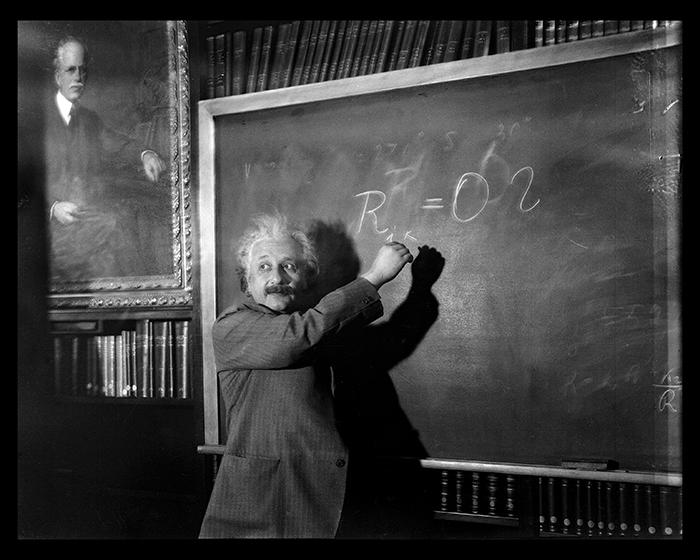## What Counts As Evidence in Mathematics?The ultimate form of argument, and for some, the most absolute form of truth, is mathematical proof. But short of a conclusive proof of a theorem, mathematicians also consider evidence that might 1) disprove a thesis or 2) suggest its possible truth or even avenues for proving that it's true. But in a not-quite-empirical field, what the heck counts as evidence?

The twin primes conjecture is one example where evidence, as much as proof, guides our mathematical thinking. Twin primes are pairs of prime numbers that differ by 2 — for example, 3 and 5, 11 and 13, and 101 and 103 are all twin prime pairs. The twin primes conjecture hypothesizes that there is no largest pair of twin primes, that the pairs keep appearing as we make our way toward infinity on the number line.

The twin primes conjecture is not the Twin Primes Theorem, because, despite being one of the most famous problems in number theory, no one has been able to prove it. Yet almost everyone believes it is true, because there is lots of evidence that supports it.

For example, as we search for large primes, we continue to find extremely large twin prime pairs. The largest currently known pair of twin primes have nearly 400,000 digits each. And results similar to the twin primes conjecture have been proved. In 2013, Yitang Zhang shocked the mathematical world by proving that there are infinitely many prime number pairs that differ by 70 million or less. Thanks to a subsequent public "Polymath" project, we now know that there are infinitely many pairs of primes that differ by no more than 246. We still haven't proved that there are infinitely many pairs of primes that differ by 2 — the twin primes conjecture — but 2 is a lot closer to 246 than it is to infinity.

This starts to get really complicated once you leave the relatively straightforward arithmetical world of prime numbers behind, with its clearly empirical pairs and approximating conjectures, and start working with computer models that generate arbitrarily large numbers of mathematical statements, all of which can be counted as evidence.

Patrick Hanner, the author of this article, gives what seems like a simple example: are all lines parallel or intersecting? Then he shows how the models one can use to answer this question vary wildly based on their initial assumptions, in this case, whether one is considering lines in a single geometric plane or lines in an n-dimensional geometric space. As always in mathematics, it comes back to one's initial set of assumptions; you can "prove" (i.e., provide large quantities of evidence for) a statement with one set of rules, but that set of rules is not the universe.